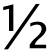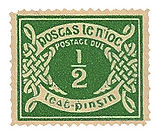# One half

﻿
One half

 One halfprefixes hemi- (from Greek) semi-/demi- (from Latin) Binary 0.1 or 0.011111111111... Decimal 0.5 or 0.499999999999... Hexadecimal 0.8 or 0.7FFFFFFFFFFF... Continued fraction [0; 1, 1] or [0; 2] Single-precision floating point 3F000000 (hex) = 00111111000000000000000000000000 (binary)

One half is the irreducible fraction resulting from dividing one by two (½), or any number by its double; multiplication by one half is equivalent to division by two. It appears often in mathematical equations, recipes, measurements, etc. Half can also be said to be one part of something divided into two equal parts.

For instance, the area S of a triangle is computed

S = ½ × base × perpendicular height.

One half also figures in the formula for calculating figurate numbers, such as triangular numbers and pentagonal numbers:

½ × n [(s - 2) n - (4 - s)]

and in the formula for computing magic constants for magic squares

M2(n) = ½ × [n (n2 + 1 )].

The Riemann hypothesis states that every nontrivial complex root of the Riemann zeta function has a real part equal to 1/2.

One half has two different decimal expansions, the familiar 0.5 and the recurring 0.49999999... It has a similar pair of expansions in any even base. It is a common trap to believe these expressions represent distinct numbers: see the proof that 0.999... equals 1 for detailed discussion of a related case.

## Particularities in writing and languagePostal stamp, Ireland, 1940: one halfpenny postage due.

½ is also one of the few fractions to get a key of its own on typewriters (see fractions). It also gets its own point in some early extensions of ASCII at 171; and in Unicode, it gets its own code point at U+00BD (decimal 189) in the C1 Controls and Latin-1 Supplement block, and a cross-reference in the Number Forms block, which contains some other fractions.

One half is as well one of the few fractions which is commonly expressed in natural languages by suppletion rather than regular derivation; compare English one half with regular formations like one sixth from six.

Wikimedia Foundation. 2010.

### Look at other dictionaries:

• one-half — n. one of two equal parts of a divisible whole; a half; a century and one half. Syn: half. [WordNet 1.5] …   The Collaborative International Dictionary of English

• one-half — noun one of two equal parts of a divisible whole (Freq. 7) half a loaf half an hour a century and one half • Syn: ↑half • Hypernyms: ↑common fraction, ↑ …   Useful english dictionary

• one half — See half …   Ballentine's law dictionary

• ONE HALF — …   Useful english dictionary

• one half of the world does not know how the other half lives — Cf. 1532 RABELAIS Pantagruel II. xxxii. la moytié du monde ne sçait comment l’autre vit, one half of the world knows not how the other lives. 1607 J. HALL Holy Observations xvii. One half of the world knowes not how the other liues: and therefore …   Proverbs new dictionary

• one-half period — pusperiodis statusas T sritis automatika atitikmenys: angl. half cycle; half cycle; half period; one half period; semiperiod vok. halbe Periode, f; Halbperiode, f rus. полупериод, m pranc. demi cycle, m; demi période, f …   Automatikos terminų žodynas

• one-half period rectification — vienpusis lyginimas statusas T sritis fizika atitikmenys: angl. half wave rectification; one half period rectification; single wave rectification vok. Einweggleichrichtung, f rus. однополупериодовое выпрямление, n pranc. redressement à simple… …   Fizikos terminų žodynas

• One-half-cloth — Полутканевый (о переплёте) …   Краткий толковый словарь по полиграфии

• six of one, half a dozen of the other — see ↑six • • • Main Entry: ↑dozen six of one, half (a) dozen of the other (US) (or chiefly Brit six of one and half a dozen of the other) informal used to say that you do not see any real difference between two possible choices …   Useful english dictionary

• six of one, half a dozen of the other — six of one, (and) half a dozen of the other two things are almost the same or equal. I also compared the two stereos, and in most respects it s six of one, half a dozen of the other. Etymology: based on the idea that half a dozen (= half of 12)… …   New idioms dictionary